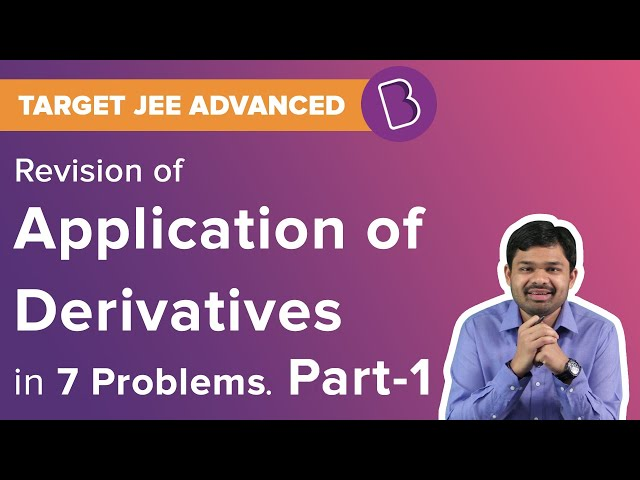# Application of Derivatives Class 12 Notes Chapter 6

## What are Derivatives?

Derivatives are referred to the situation when a quantity p varies with respect to another quantity q, fulfilling a condition p =f (q) and dp/dq (or f ‘ (q)) represents the change of rate of p with respect to q and dp/dq where q =

$$\begin{array}{l}q_{0}\end{array}$$
or f ‘ (
$$\begin{array}{l}q_{0}\end{array}$$
) represents the rate of change of p with respect to q at q =
$$\begin{array}{l}q_{0}\end{array}$$
.

Students can refer to the short notes and MCQ questions along with separate solution pdf of this chapter for quick revision from the links below:### Chain Rule

Let us consider two variables, p and q, which vary with respect to the presence of another variable r, which means p = f(r) and q = g(r), then according to the chain rule,

$$\begin{array}{l}\frac{\partial q}{\partial p} = \frac{\partial q}{\partial r}/\frac{\partial p}{\partial r}, if \frac{\partial p}{\partial r}\neq 0\end{array}$$

### Increasing and Decreasing Function

A Function p can be said to increase when:

• We can see an increase on an interval (a,b) if
$$\begin{array}{l}x_{1}<x_{2}\end{array}$$
in (a,b) =>
$$\begin{array}{l}p(x_{1})<p(x_{2})\end{array}$$
for all
$$\begin{array}{l}x_{1}, x_{2}\epsilon (a,b)\end{array}$$

A function is said to be decreasing when:

• if
$$\begin{array}{l}x_{1}<x_{2}\end{array}$$
in (a,b) when:
$$\begin{array}{l}x_{1}>x_{2}\end{array}$$
for all
$$\begin{array}{l}x_{1}, x_{2}\epsilon (a,b)\end{array}$$

### Constant

The constant term in (a,b) if p(x)= q for all

$$\begin{array}{l} x \epsilon (a,b)\end{array}$$
and q is a constant

### Equation of Tangent

The equation of a tangent is given at a point

$$\begin{array}{l}(x_{0}, y_{0})\end{array}$$
to the curve y =f(x) is represented by
y-yo=[dy/dx](xo,yo)(x-xo)

### Critical point

The point q inside the domain of a function f at which f ‘ (q) =0 or f cannot be differentiable is referred to as the critical point of f.

 Also Access NCERT Solutions for Class 12 Maths Chapter 6 NCERT Exemplar for Class 12 Maths Chapter 6

### Important Questions

1. Find out the value of m if line y=mx+1, which is a tangent to the curve
$$\begin{array}{l}y^{2}=4x\end{array}$$
.
2. For the curve
$$\begin{array}{l}2y+x^{2}=3\end{array}$$
is?
1. A semicircular opening is surmounted by a window which is in the form of a rectangle. The total perimeter of the window is 50 m. Find the dimensions of the window to admit maximum light through the whole opening.
1. A point on the hypotenuse of a triangle is at a distance p and q from the sides of the triangle.
1. For what values of a the function f given by f(x) =
$$\begin{array}{l}x^{2}\end{array}$$
+ ax + 1 is increasing On [1, 2]?

 Derivatives Derivative of a Function-Calculus

## Frequently Asked Questions on CBSE Class 12 Maths Notes Chapter 6 Application of Derivations

Q1

### What is the chain rule?

The chain rule states that the derivative of f(g(x)) is f'(g(x))⋅g'(x).

Q2

### What are the uses of derivations?

1. Determine the velocity of a body 2. Solve distance and displacement problems 3. Change in population size

Q3

### What is the critical point?

Critical points are places where the derivative of a function is either zero or undefined. These critical points are places on the graph where the slope of the function is zero.